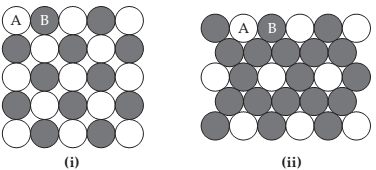### Create an Account

Home / Questions / Two patterns of packing for two different spheres are shown here. For each structure (a) d...

# Two patterns of packing for two different spheres are shown here. For each structure (a) draw the two-dimensional unit cell

Two patterns of packing for two different spheres are shown here. For each structure (a) draw the two-dimensional unit cell; (b) determine the angle between the lattice vectors, γ, and determine whether the lattice vectors are of the same length or of different lengths; and (c) determine the type of two-dimensional lattice (from Figure 12.4).Aug 23 2021 View more View LessSubscribe To Get Solution